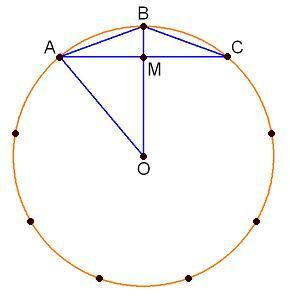You may also likeCoke Machine

The coke machine in college takes 50 pence pieces. It also takes a certain foreign coin of traditional design...At a Glance

The area of a regular pentagon looks about twice as a big as the pentangle star drawn within it. Is it?Cosines Rule

Three points A, B and C lie in this order on a line, and P is any point in the plane. Use the Cosine Rule to prove the following statement.

Sine and Cosine for Connected Angles

Age 14 to 16Challenge Level

The diagram shows a nine-point pegboard. We'll take the board radius as one (in other words it's a unit circle).Looking at triangle $OAM$, why is $AM$ equal to $\sin40^\circ$?

Looking at triangle $OAB$, why is $AB$ equal to $2\sin20^\circ$?

Looking at triangle $ABM$, why is $AM$ also equal to $AB\cos20^\circ$ which works out as $2\sin20^\circ$ $\cos20^\circ$

So it looks like the same $AM$ length value can be calculated by using $\sin40^\circ$

and also calculated by using $2\sin20^\circ\cos 20^\circ$

Now switch to a $10$ point pegboard and find the two ways to calculate the $AM$ length on that board.

How about on a $12$ point board? What general result is emerging?

Draw a diagram and use it to provide reasoning which accounts for that general result.

For printable sets of circle templates for use with this activity, please see Printable Resources page.

Many thanks to Geoff Faux who introduced us to the merits of the 9 pin circular geo-board.

The boards, moulded in crystal clear ABS that can be used on an OHP (185 cm in diameter), together with a teacher's guide, are available from Geoff at Education Initiatives